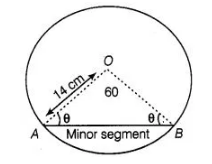# Find the area of the minor segment

Question:

Find the area of the minor segment of a circle of radius 14 cm, when the angle of the corresponding sector is 60°.Solution:

Given that, radius of circle (r) = 14 cm

and angle of the corresponding sector i.e., central angle (θ) = 60°

Since, in ΔAOB, OA = OB = Radius of circle i.e., ΔAOB is isosceles.

$\Rightarrow \quad \angle O A B=\angle O B A=\theta$

Now, in $\triangle O A B \quad \angle A O B+\angle O A B+\angle O B A=180^{\circ}$

[since,sum of interior angles of any triangle is $180^{\circ}$ ]

$\Rightarrow \quad 60^{\circ}+\theta+\theta=180^{\circ}$  [given, $\angle A O B=60^{\circ}$ ]

$\Rightarrow \quad 2 \theta=120^{\circ}$

$\Rightarrow \quad \theta=60^{\circ}$

i.e. $\angle O A B=\angle O B A=60^{\circ}=\angle A O B$

Since, all angles of $\triangle A O B$ are equal to $60^{\circ} i . e ., \triangle A O B$ is an equilateral triangle.

Also, $O A=O B=A B=14 \mathrm{~cm}$

So, Area of $\triangle O A B=\frac{\sqrt{3}}{4}$ (side) $^{2}$

$=\frac{\sqrt{3}}{4} \times(14)^{2}\left[\because\right.$ area of an equilaterai triangle $\left.=\frac{\sqrt{3}}{4}(\text { side })^{2}\right]$

$=\frac{\sqrt{3}}{4} \times 196=49 \sqrt{3} \mathrm{~cm}^{2}$